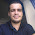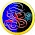## Friday, January 3, 2014

### Backtracking: Solve Sudoku in Java

This post demonstrates solving Sudoku using Backtracking. Algorithm and a working code sample is given. The code is meant to be self-explanatory, try to understand the code, it 's actually simple.

References:

Backtracking: http://en.wikipedia.org/wiki/Backtracking
Sudoku: http://en.wikipedia.org/wiki/Sudoku

Pre-condition:

# Read input grid (this is in matrix form)
# Grid has 9x9 cells. Cell is represented by (x,y)
# Each cell contains a value from 1 to 9.
# Empty cells are contain the value '0'

Algorithm:

1# solve(cell)
2# If cell not empty, call solve(nextCell)
3# If cell is empty (grid[cell.x][cell.y] == 0)
4# Add value v (v belongs to 1-9)
5# Check if v is valid for cell
6# if not valid, continue to #4
7# If valid, call solve(nextCell)
8# If sudoku solved, return true
9# Else return false

Explanation:

• Create a function solve(currentCell) which takes a cell and returns true/false based on whether the Sudoku is solved or not.
• Remember if the cell already has a value, there is nothing to do.
• In each call our job is to find a suitable value from 1-9 for the cell, which will solve the sudoku.
• If we cannot find such value, we backtrack.
• First focus on simple conditions:
• If currentCell is null, we have reached the end. Return true. (We have solved the problem)
• If currentCell already has a value, no-op (Nothing to do here, call next)
• Now we know currentCell is empty, try each value from 1-9 (checkout the for loop in the code)
• For each value, check if its valid, then put the value else just keep putting next value.
• See if sudoku is solved; if solved, just return true, else return false
• If for loop ends, this means we did not find any valid value for currentCell, something is wrong in the previous value,
• Reset the value to 0, and just return false.

Working Code:
``````
package com.example;

public class Sudoku {

// dimension of input
static int N = 9;

// sample input
static int grid[][] = { { 3, 0, 6, 5, 0, 8, 4, 0, 0 }, //
{ 5, 2, 0, 0, 0, 0, 0, 0, 0 }, //
{ 0, 8, 7, 0, 0, 0, 0, 3, 1 }, //
{ 0, 0, 3, 0, 1, 0, 0, 8, 0 }, //
{ 9, 0, 0, 8, 6, 3, 0, 0, 5 }, //
{ 0, 5, 0, 0, 9, 0, 6, 0, 0 }, //
{ 1, 3, 0, 0, 0, 0, 2, 5, 0 }, //
{ 0, 0, 0, 0, 0, 0, 0, 7, 4 }, //
{ 0, 0, 5, 2, 0, 6, 3, 0, 0 } };

/**
* Class to abstract the representation of a cell. Cell => (x, y)
*/
static class Cell {

int row, col;

public Cell(int row, int col) {
super();
this.row = row;
this.col = col;
}

@Override
public String toString() {
return "Cell [row=" + row + ", col=" + col + "]";
}
};

/**
* Utility function to check whether @param value is valid for @param cell
*/

static boolean isValid(Cell cell, int value) {

if (grid[cell.row][cell.col] != 0) {
throw new RuntimeException(
"Cannot call for cell which already has a value");
}

// if v present row, return false
for (int c = 0; c < 9; c++) {
if (grid[cell.row][c] == value)
return false;
}

// if v present in col, return false
for (int r = 0; r < 9; r++) {
if (grid[r][cell.col] == value)
return false;
}

// if v present in grid, return false

// to get the grid we should calculate (x1,y1) (x2,y2)
int x1 = 3 * (cell.row / 3);
int y1 = 3 * (cell.col / 3);
int x2 = x1 + 2;
int y2 = y1 + 2;

for (int x = x1; x <= x2; x++)
for (int y = y1; y <= y2; y++)
if (grid[x][y] == value)
return false;

// if value not present in row, col and bounding box, return true
return true;
}

// simple function to get the next cell
// read for yourself, very simple and straight forward
static Cell getNextCell(Cell cur) {

int row = cur.row;
int col = cur.col;

// next cell => col++
col++;

// if col > 8, then col = 0, row++
// reached end of row, got to next row
if (col > 8) {
// goto next line
col = 0;
row++;
}

// reached end of matrix, return null
if (row > 8)
return null; // reached end

Cell next = new Cell(row, col);
return next;
}

// everything is put together here
// very simple solution
// must return true, if the soduku is solved, return false otherwise
static boolean solve(Cell cur) {

// if the cell is null, we have reached the end
if (cur == null)
return true;

// if grid[cur] already has a value, there is nothing to solve here,
// continue on to next cell
if (grid[cur.row][cur.col] != 0) {
// return whatever is being returned by solve(next)
// i.e the state of soduku's solution is not being determined by
// this cell, but by other cells
return solve(getNextCell(cur));
}

// this is where each possible value is being assigned to the cell, and
// checked if a solutions could be arrived at.

// if grid[cur] doesn't have a value
// try each possible value
for (int i = 1; i <= 9; i++) {
// check if valid, if valid, then update
boolean valid = isValid(cur, i);

if (!valid) // i not valid for this cell, try other values
continue;

// assign here
grid[cur.row][cur.col] = i;

// continue with next cell
boolean solved = solve(getNextCell(cur));
// if solved, return, else try other values
if (solved)
return true;
else
grid[cur.row][cur.col] = 0; // reset
// continue with other possible values
}

// if you reach here, then no value from 1 - 9 for this cell can solve
// return false
return false;
}

public static void main(String[] args) {
boolean solved = solve(new Cell(0, 0));
if (!solved) {
System.out.println("SUDOKU cannot be solved.");
return;
}
System.out.println("SOLUTION\n");
printGrid(grid);
}

// utility to print the grid
static void printGrid(int grid[][]) {
for (int row = 0; row < N; row++) {
for (int col = 0; col < N; col++)
System.out.print(grid[row][col]);
System.out.println();
}
}
}

```
```

#### 3 comments :

1.2.Hi, this is a grate work, but there a fault, if for example the entry is wrong, your algorithme will not detect this. I explain, for example if the first ine contain two occuration of the number 3, the algo. will not detect the fault and will continue resolution with the wrong entry.
So, you should test if the is ok or not in the begning :)

3.